# Quiz 11: Managing Capacity and Demand

Business

Following constraints are needed to be taken into consideration before making a schedule: • Each controller will work in 8-hour shift and each controller must be allowed 2 consecutive days off. • Each controller would be required to work for exactly 5 days in a week. • The ratio of total operations to the available controllers must not exceed 16. • There are three shifts that run from 00:01 to 8:00, 08:01 to 16:00 and from 16:01 to 24:00. The table given below shows the number of operations that are required for each day: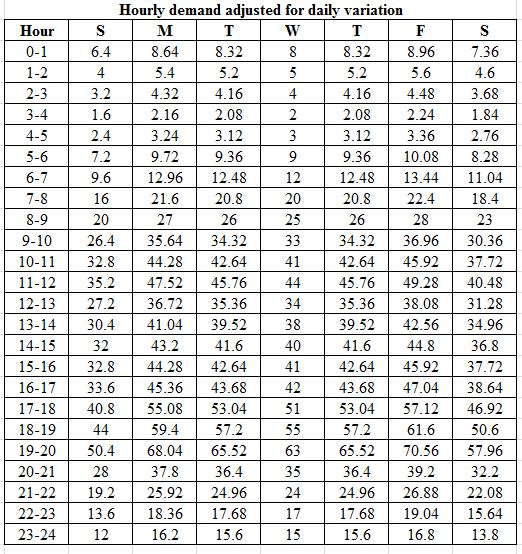The subsequent number of operators required in order to perform the required number of operations as given in the table shown above are given below: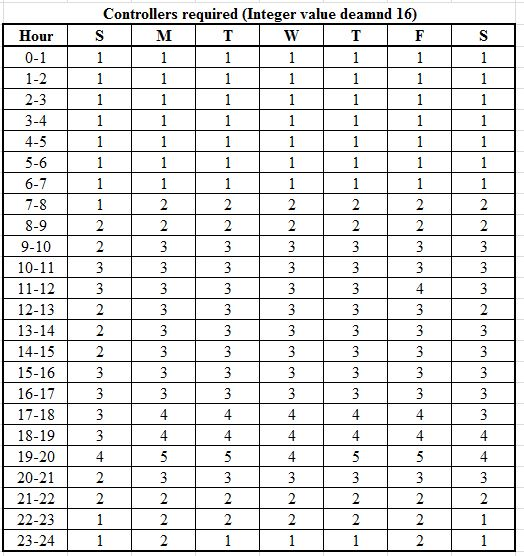The minimum number of controllers required can be summarized as per the shift hours as shown in the table given below: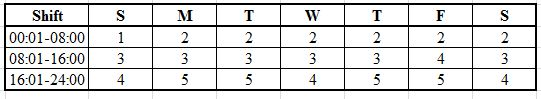The minimum number of controllers that are required after keeping into consideration various constraints can be calculated by using integer linear programming. Let the variables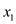,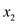,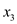,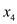,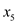,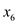and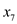, represent the minimum number of staffs available on a given day. Thus, the objective function will be, Minimize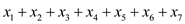Subject to, Sunday constraint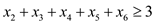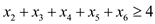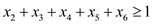Monday constraint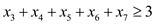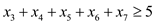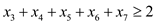Tuesday constraintWednesday constraintThursday constraintFriday constraintSaturday constraintThe above linear programming can be solved by using solver in excel. Input the decision variables, objective function and the constraints as shown below: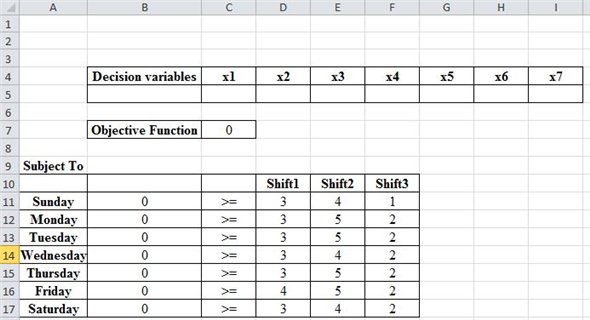The formulas that have been used in the above given excel sheet are shown below: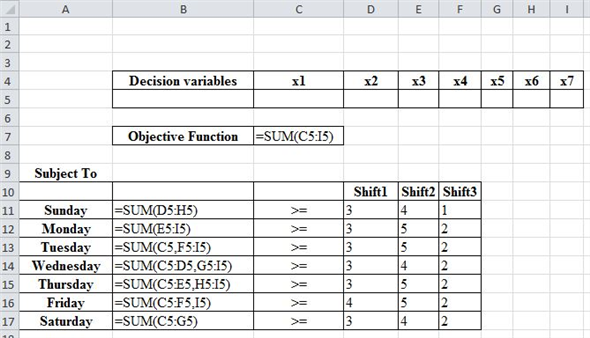Select the objective function in the excel sheet and use solver. Input the constraints for shift 1, select the minimize function and make all the variables as integer as shown in the figure given below: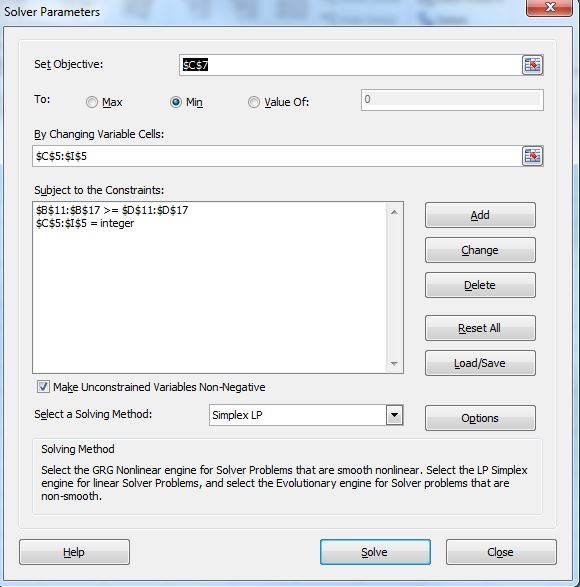Click on the solve button in order to obtain the values for the decision variables as shown below: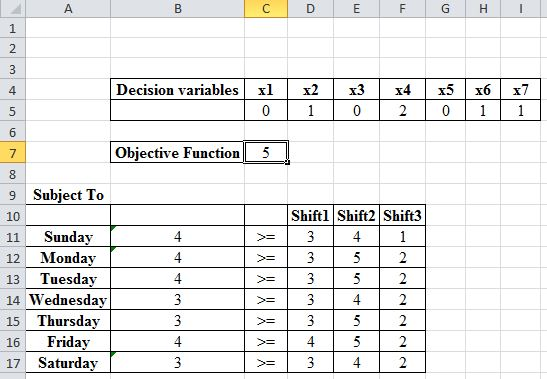Thus, the minimum numbers of controllers that are required in the first shift are 5. Now, make all the decision variables equal to zero and select the objective function. Select solver in the excel sheet. In the constraints delete the constraints related with shift 1 and now select the constraints that are related with shift 2 as shown in the figure given below: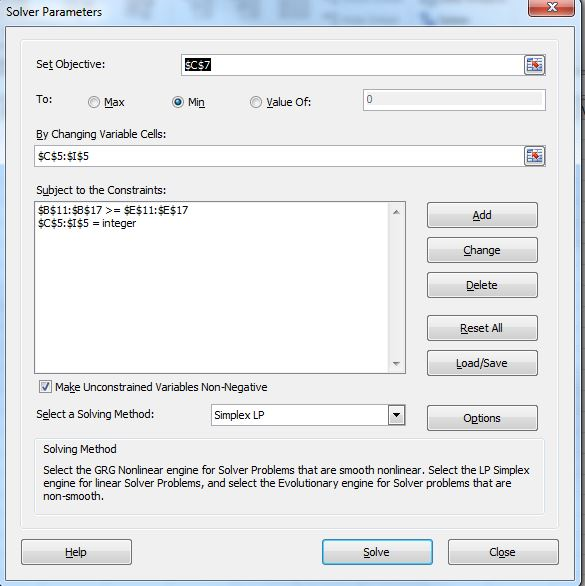Click on the solve button in order to obtain the number of controllers required for shift 2. The figure given below shows the number of controllers that are required for shift 2: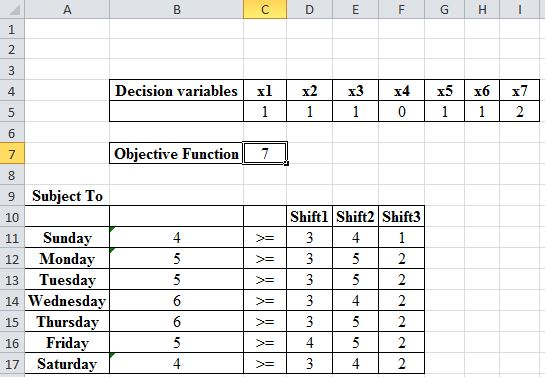Thus, the numbers of controllers that are required for shift 2 are 7. Similarly, calculate the number of controllers required for shift 3 by deleting the previous constraint and adding the constraint related with shift 3. After solving in the excel the number of controllers as shown below are obtained: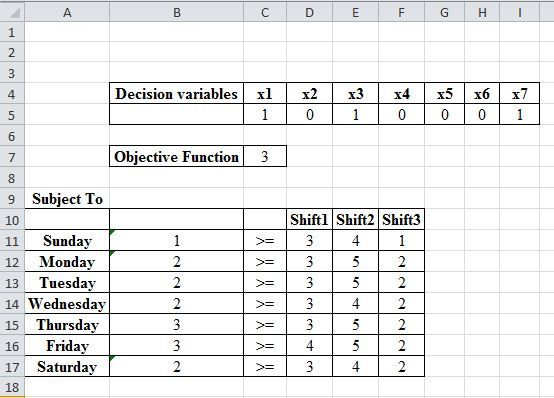Thus, 3 controllers are required in shift 3. Thus, a minimum of 15 controllers are required to work in three shifts after taking into consideration various constraints.

Sequoia Airlines faces the problem of planning the optimal use of its resources during the next six months.  It must hire new employees and develop them through three stages of training, first as trainees, then as junior flight attendants, and finally as full flight attendants.  Moreover, Sequoia must meet staff requirements for all flights during the training period.  This problem is analyzed using total personnel cost as the selection criterion. Other resources in addition to the available personnel create constraints that must be considered when hiring and training new employees.  We will solve the problem by using linear programming and then follow up with an analysis of the objective function and the constraints and assumptions on which they are based.  We will also offer an interpretation of the results. Because cost is the only criterion we will use to solve this problem, the objective function is straightforward.  One assumption is noteworthy:  we assume that once an experienced attendant becomes an instructor, he or she continues to receive the instructor's salary even when there is a surplus of instructors who act as attendants.  The objective function, therefore, becomes the sum of the number of employees in each class during each period multiplied by the appropriate wage. Minimize: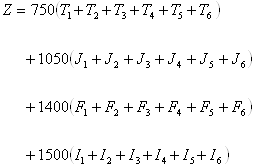We must also deal with several resource constraints.  First, we must meet the requirement for hours of in-flight attendant service.  We assume that a surplus instructor works the same number of hours as an experienced attendant does.Next, we must satisfy the constraint that requires the total number of hours for junior attendants to be less than or equal to 25 percent of the total attendant hours in each month.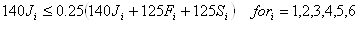Also, there must be one instructor for every five trainees.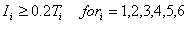The remaining constraints deal with the relationships between the status of employees during the current and prior periods.  In this case we make assumptions that pertain to the initial staffing levels for the positions of junior attendant, full attendant, and instructor.We see a total of 42 constraints in the following computer model. Min 1050J1+ 1050J2+ 1050J3+ 1050J4+ 1050J5+ 1050J6+ 1400F1+ 1400F2+ …Subject to(1) 140J1+ 125F1+ 125S1 = 14000(2) 140J2+ 125F2+ 125S2 = 16000(3) 140J3+ 125F3+ 125S3 = 13000(4) 140J4+ 125F4+ 125S4 = 12000(5) 140J5+ 125F5+ 125S5 = 18000(6) 140J6+ 125F6+ 125S6 = 20000(7) 105Jl-31.25Fl-31.25S1 = 0(8) 105J2-31.25F2-31.25S2 = 0(9) 105J3-31.25F3-31.25S3 = 0(10) 105J4-31.25F4-31.25S4 = 0(11) 105J5-31.25F5-31.25S5 = 0(12) 105J6-31.25F6-31.25S6 = 0(13) 1I1-.2T1 = 0(14) 112-.2T2 = 0(15) 113-.2T3 = 0(16) 114-.2T4 = 0(17) 115-.2T5 = 0(18) 116-.2T6 = 0(19) 1J1 = 8(20) 1F1 = 119(21) 111 = 6(22) -111+ 1Sl+.2T1 = 0(23) -112+ 1S2+.2T2 = 0(24) -113+ 1S3+.2T3 = 0(25) -114+ 1S4+.2T4 = 0(26) -115+ 1S5+.2T5 = 0(27) -116+ 1S6+.iT6 = 0(28) 1J2-.8T1 = 0(29) 1J3-.8T2 = 0(30) 1J4-.8T3 = 0(31) 1J5-.8T4 = 0(32) 1J6-.8T5 = 0(33) -.95J1-.92F1+ 1F2 = 0(34) -.95J2-.92F2+ 1F3 = 0(35) -.95J3-.92F3+ 1F4 = 0(36) -.95J4-.92F4+ 1F5 = 0(37) -.95J5-.92F5+ 1F6 = 0(38) -.005F1-.95I1+ 112 = 0(39) -.005F2-.9512+ 113 = 0(40) -.005F3-.9513+ 114 = 0(41) -.005F4-.9514+ 115 = 0(42) -.005F5-.9515+ 116 = 0

Consider the given data showing the expected number of walk-ins each day in an outpatient clinic: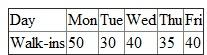a. The clinic was employing 5 physicians each having the capacity to examine 15 patients per day. Therefore, maximum number of appointments that can be handled by the clinic is 75. Hence, if the clinic wants to smooth out the demand for the entire week, then the maximum number of appointments that should be scheduled for each day is 75. The numbers of patients which are required per day to smooth the demand are calculated by subtracting the walk-in patients from the daily physician capacity. The final table is shown below: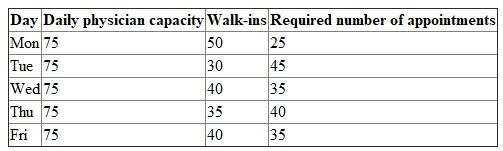b. The appointments should not be scheduled at their maximum level , because the walk-ins values given in the table only represent the forecasted values. The actual arrival of patients would vary due to customer-induced variability. Furthermore, it is obvious that the capacity of a server must always exceed the demand. This would retain some slack time in the system which would further help in reducing the prolonged waiting time of the patients. c. Few appointments should be made at the very beginning of the day in order to safeguard the physicians from waiting. It would help to reduce the idle time of the physicians. Besides this, most of the appointments should be scheduled in the morning and afternoon hours to avoid excessive waiting of the patients. Thus, it would help to reduce the waiting time of the customers in the system.